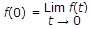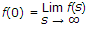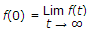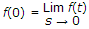# Electronics and Communication Engineering - Networks Analysis and Synthesis

1.

Bandwidth will be

 A. a.b B. a + b C. a D. b

Explanation:

No answer description available for this question. Let us discuss.

2.

In carbon resistor colour code, the fourth band indicates tolerance.

 A. True B. False

Explanation:

No answer description available for this question. Let us discuss.

3.

For any given two port network, any parameter set can be determined from measurements made at the two ports.

 A. True B. False

Explanation:

No answer description available for this question. Let us discuss.

4.

For 200V r.m.s. value triangular wave, the peak voltage will be

 A. 200 V B. 222 V C. 280 V D. 346 V

Explanation:

No answer description available for this question. Let us discuss.

5.

According to initial value theorem which one is correct

1.2.3.4.A. 1, 4 B. 2, 3 C. 4, 2 D. 1, 2